Next: MINIMUM UNSATISFYING LINEAR SUBSYSTEM Up: Mathematical Programming Previous: MINIMUM RELEVANT VARIABLES IN   Index

### MAXIMUM SATISFYING LINEAR SUBSYSTEM

• INSTANCE: System Ax=b of linear equations, where A is an integer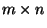-matrix, and b is an integer m-vector.

• SOLUTION: A rational n-vector.

• MEASURE: The number of equations that are satisfied by x.

• Good News: Approximable within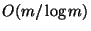.

• Bad News: Not approximable within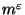for someand .

• Comment: For any prime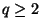the problem over GF[q] is approximable within q, but is not approximable within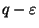for any, even if the number of variables in each equation is exactly three. When there are at most two variables in each equation the problem over GF is approximable within 1.383 but is not approximable within 1.0909 ; not approximable within 1.0030 if the number of occurrences of any variable is bounded by 3 . When there are at most two variables in each equation the problem over GF[q] for any primeis approximable within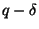for some(dependent on q) but not approximable within 70/69for any. When there are exactly k variables in each equation and the number of equations isthe problem over GF[q] for any primeadmits a randomized PTAS .

The variation in which the system consists of relations (> or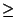) is APX-complete and approximable within 2 . If the variables are restricted to assume only binary values, the problem is harder to approximate than MAXIMUM INDEPENDENT SET. Approximability results for even more variants of the problem can be found in .Next: MINIMUM UNSATISFYING LINEAR SUBSYSTEM Up: Mathematical Programming Previous: MINIMUM RELEVANT VARIABLES IN   Index
Viggo Kann
2000-03-20